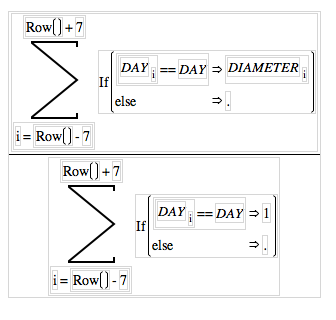Our World Statistics Day conversations have been a great reminder of how much statistics can inform our lives. Do you have an example of how statistics has made a difference in your life? Share your story with the Community!
Choose Language Hide Translation Bar
Highlighted

## Calculate moving average for By-Groups

I'm trying to calculate a moving average for a data-set containing several rounds of the continuous data. That is, I want a calculated average for every data-point, including the first and last. The average should be 7 steps in either direction, except for either end, where non-existing data beyond the 7 step window should simply ignored. Of course I don't want data form different By-Groups to be included in the same average. I was hoping to use the "Col Mean" function, but I can't figure out how to make the average "move". Any suggestions?

BR
Jesper
1 ACCEPTED SOLUTION

Accepted Solutions
HighlightedJeff_PerkinsonCommunity Manager

## Re: Calculate moving average for By-Groups

Hi Jesper,

You can use the Summation function with an If() condition to do this.

Here's a picture of what it would look like in the formula editor.And here's the text version:

Summation(

i = Row() - 7,

Row() + 7,

If( :DAY == :DAY

:DIAMETER,

.

)

) / Summation( i = Row() - 7, Row() + 7, If( :DAY == :DAY, 1, . ) )

Hope that helps.

-Jeff

-Jeff
4 REPLIES 4
Highlighted

## Re: Calculate moving average for By-Groups

Do you have ETS licenced?  If so, there is functionality within PROC EXPAND to calculate moving averages

Highlighted

## Re: Calculate moving average for By-Groups

I do have access to EST, but I have never used it. I was hoping to do it directly in the formula editor.

BR
Jesper
HighlightedJeff_PerkinsonCommunity Manager

## Re: Calculate moving average for By-Groups

Hi Jesper,

You can use the Summation function with an If() condition to do this.

Here's a picture of what it would look like in the formula editor.And here's the text version:

Summation(

i = Row() - 7,

Row() + 7,

If( :DAY == :DAY

:DIAMETER,

.

)

) / Summation( i = Row() - 7, Row() + 7, If( :DAY == :DAY, 1, . ) )

Hope that helps.

-Jeff

-Jeff
Highlighted

## Re: Calculate moving average for By-Groups

That was exactly what I needed. Great! Thank you.

BR
Jesper
Article Labels

There are no labels assigned to this post.